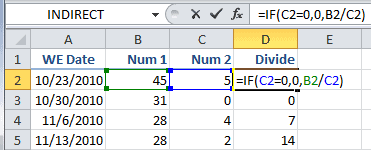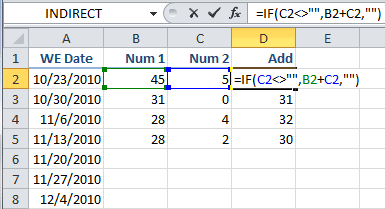The IF Function in Excel

The IF Function is used to evaluate a logical test, then if TRUE do something or if FALSE do something else. A familiar construct. The IF Function has three arguments:

IF(logical_test, [value_if_true], [value_if_false])

Argument 1

The first argument, logical_test, is required. It’s any value or expression that can be evaluated to TRUE or FALSE. This argument can use any comparison calculation operator. There are four types:

• Arithmetic operators ( +   –   *   /   %   ^ )
• Comparison operators ( =   >   <   >=   <=   >< )
• Text concatenation operator ( & )
• Reference operators (colon, comma, space )

Argument 2

The second argument, value_if_true, is optional. This value is returned if the logical_test argument evaluates to TRUE.

I didn’t know this second argument was OPTIONAL, but nevertheless you can omit this argument by placing a comma after the first argument, followed by nothing. An example would be =IF(A1= 5,). But here’s the weird part, if the second argument is omitted and TRUE, the function returns a zero (0), and if the second argument is omitted and FALSE the function returns FALSE.

When TRUE = 1 and FALSE = 0 then everything is right with the Excel world. When TRUE = 0 then things are messed up. I think they messed up here. Although how many people omit the second argument in an IF statement? But I digress.

Argument 3

The third argument, value_if_false, is optional. This value is returned if the logical_test argument evaluates to FALSE.

Two Common Uses for the IF Function

Division by zero is undefined mathematically so Excel provides a glaring #DIV/0! when that happens. And sometimes we don’t want to show the result of a formula unless there’s some actual data to calculate.

Avoid Division by Zero

The intent for column D is to divide Num1 by Num 2, but avoid division by zero. The IF function is looking to see if Num 2 is zero (0) and if so, then zero(0)  is returned, otherwise the calculation is done (Num 1 / Num 2). The formula in cell D2 is =IF(C2=0,0,B2/C2).Knowing what I do now about omitting the second argument, this formula could be re-written as =IF(C2=0,,B2/C2) with the same result. However, not a very good practice.

More commonly this formula is written differently as =IF(C2<>0,B2/C2,0) with the same result; doing the calculation if Num 2 is not zero (0) and returning a zero (0) when it is.

Calculate or Leave Blank

Many times formulas extend beyond currently entered data. This is where the IF function can be useful. The formula in cell D2 is =IF(C2<>””,B2+C2,””), which adds Num 1 and Num 2 if Num2 is not blank, and returns a blank (“”) otherwise.This formula could also be written =IF(C2=””,””,B2+C2) which reads like this: if C2 is blank, then return a blank, otherwise add B2 and C2. Both of these formulas give the same result.13 thoughts on “The IF Function in Excel”

1.Dennis Taylor

Here are two other options to consider:

First, use the ISBLANK function for greater clarity: =IF(ISBLANK(C2),0,B2/C2)

Second, use the new IFERROR function; it’s more direct: =IFERROR(B2/C2,0)

1.Gregory

ISBLANK would be a great deal clearer, but I’m probably going to use “” to save some time.

The IFERROR function is nice but you have to be careful that the file doesn’t get shared with someone using an older version of Excel.

2.Jerry

Calculate Leave
Whats wrong with this: =IF([Status],”IF([FT]=15),IF([PT]=10)”,”IF([CN]=0)”)

Status (FT,PT,CN) 15 Days for FT
10 Days for PT
0 For CN

1.Gregory

The first argument is a logical test, which is: Any value or expression that can be evaluated to TRUE or FALSE. I couldn’t get past the first argument because I don’t know what [Status] is supposed to reference.

If I change the first argument to something like A1=0, and cell A1 is in fact zero (0), then the formula returns IF([FT]=15),IF([PT]=10) as a text value. If I put a number, like 1, in cell A1 the formula returns IF([CN]=0) as a text value.

3.Kathy Stohlmann

Hey there, I’m trying to create an IF statement and I’m not succeeding. I’m self taught so that’s part of the problem. If I write out the statement will someone help me with the IF statement, please

1.Gregory

Let er rip, tater chip. I mean, sure, write out what you want to do with the IF statements.

4.Nathan

Hi,

Ive made a sequence in excel and im trying to make an if statement where if the cell infront of it = 1 then I want it to be blank. But if it doesn’t equal one I don’t want it to change at all.. What symbol do I use?

1.Gregory

Assume the cell you are referring to is A1. The formula would be =IF(A1=””,””,A1) where if cell A1 is blank (which is two quote marks with no space) “” then you enter a blank (“”) else use the number in the cell (A1).

5.Liam

Hi,

I want to put in place a formula which divides two figures by one another to help me find the percentages, this is to be used in a professional basis.

For example I have formula’s set which count how many cells have writing in so let’s say \$B\$4: =COUNTIF(B8:B172088,”*”) & \$B\$5: =COUNTIF(C8:C123409,”*”) … I need to this formula to show a 0 … =B4/(B4+B5) … When I put this in a cell it comes up with #DIV/0! but I need the cell to show 0 until I input information, the formula works when information is applied but I need it to show 0 to make it look more presentable. Can you help me with this?

1.Gregory

The formula you want is to see if the divisor is zero and if so enter a blank, otherwise do the calculation. Instead of =B4/(B4+B5) try this out:

=IF(B4+B5=0,””,B4/(B4+B5))

6.Ian

Hello, i’m trying to use the following formula in 4 cells next to each other, the K15 cell has a data validation in it of either 25%,50%,75% or 100% but i want to return a figure only if one of the percentages are met. I think it’s something to with a true or false, but cant seem to get it to work

=IF(K15=\$R\$8,P15*\$S\$8,””)&IF(K15=\$R\$9,P15*\$S\$9,””)&IF(K15=\$R\$10,P15*\$S\$10,””)&IF(K15=\$R\$11,P15*\$S\$11,””)

1.Gregory

You would probably want something like this:

=IF(OR(K15=0.25, K15=0.5, K15=0.75, K15=1), DO THIS CALCULATION, “”)

where you would substitute your formula for DO THIS CALCULATION.